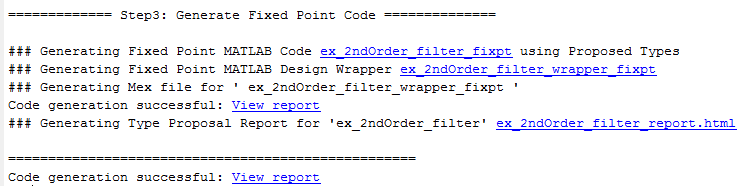## Propose Fixed-Point Data Types Based on Simulation Ranges

This example shows how to propose fixed-point data types based on simulation range data using the `codegen` function.

### Prerequisites

To complete this example, you must install the following products:

• MATLAB®

• MATLAB Coder™

• Fixed-Point Designer™

• C compiler

You can use `mex -setup` to change the default compiler. See Change Default Compiler.

### Create a New Folder and Copy Relevant Files

1. Create a local working folder, for example, `c:\ex_2ndOrder_filter`.

2. Change to the `docroot\toolbox\fixpoint\examples` folder. At the MATLAB command line, enter:

`cd(fullfile(docroot, 'toolbox', 'fixpoint', 'examples')) `

3. Copy the `ex_2ndOrder_filter.m` and `ex_2ndOrder_filter_test.m` files to your local working folder.

TypeNameDescription
Function code`ex_2ndOrder_filter.m`Entry-point MATLAB function
Test file`ex_2ndOrder_filter_test.m`MATLAB script that tests `ex_2ndOrder_filter.m`

### Set Up the Fixed-Point Configuration Object

Create a fixed-point configuration object and configure the test file name.

```fixptcfg = coder.config('fixpt'); fixptcfg.TestBenchName = 'ex_2ndOrder_filter_test'; ```

### Set Up the C Code Generation Configuration Object

Create a code configuration object to generate a C static library. Enable the code generation report.

```cfg = coder.config('lib'); cfg.GenerateReport = true;```

### Collect Simulation Ranges and Generate Fixed-Point Code

Use the `codegen` function to convert the floating-point MATLAB function, `ex_2ndOrder_filter`, to fixed-point C code. Set the default word length for the fixed-point data types to 16.

```fixptcfg.ComputeSimulationRanges = true; fixptcfg.DefaultWordLength = 16; % Derive ranges and generate fixed-point code codegen -float2fixed fixptcfg -config cfg ex_2ndOrder_filter```

`codegen` analyzes the floating-point code. Because you did not specify the input types for the `ex_2ndOrder_filter` function, the conversion process infers types by simulating the test file. The conversion process then derives ranges for variables in the algorithm. It uses these derived ranges to propose fixed-point types for these variables. When the conversion is complete, it generates a type proposal report.

### View Range Information

Click the link to the type proposal report for the `ex_2ndOrder_filter` function, `ex_2ndOrder_filter_report.html`.

The report opens in a web browser.### View Generated Fixed-Point MATLAB Code

`codegen` generates a fixed-point version of the `ex_2ndOrder_filter.m` function, `ex_2ndOrder_filter_fixpt.m`, and a wrapper function that calls `ex_2ndOrder_filter_fixpt`. These files are generated in the `codegen\ex_2ndOrder_filter\fixpt` folder in your local working folder.

```function y = ex_2ndOrder_filter_fixpt(x) %#codegen fm = get_fimath(); persistent z if isempty(z) z = fi(zeros(2,1), 1, 16, 15, fm); end % [b,a] = butter(2, 0.25) b = fi([0.0976310729378175, 0.195262145875635, 0.0976310729378175], 0, 16, 18, fm); a = fi([ 1, -0.942809041582063, 0.3333333333333333], 1, 16, 14, fm); y = fi(zeros(size(x)), 1, 16, 14, fm); for i=1:length(x) y(i) = b(1)*x(i) + z(1); z(1) = fi_signed(b(2)*x(i) + z(2)) - a(2) * y(i); z(2) = fi_signed(b(3)*x(i)) - a(3) * y(i); end end function y = fi_signed(a) coder.inline( 'always' ); if isfi( a ) && ~(issigned( a )) nt = numerictype( a ); new_nt = numerictype( 1, nt.WordLength + 1, nt.FractionLength ); y = fi( a, new_nt, fimath( a ) ); else y = a; end end function fm = get_fimath() fm = fimath('RoundingMethod', 'Floor', 'OverflowAction', 'Wrap', 'ProductMode', 'FullPrecision', 'MaxProductWordLength', 128, 'SumMode', 'FullPrecision', 'MaxSumWordLength', 128); end```

### View Generated Fixed-Point C Code

To view the code generation report for the C code generation, click the View Report link that follows the type proposal report.The code generation report opens and displays the generated code for `ex_2ndOrder_filter_fixpt.c`.